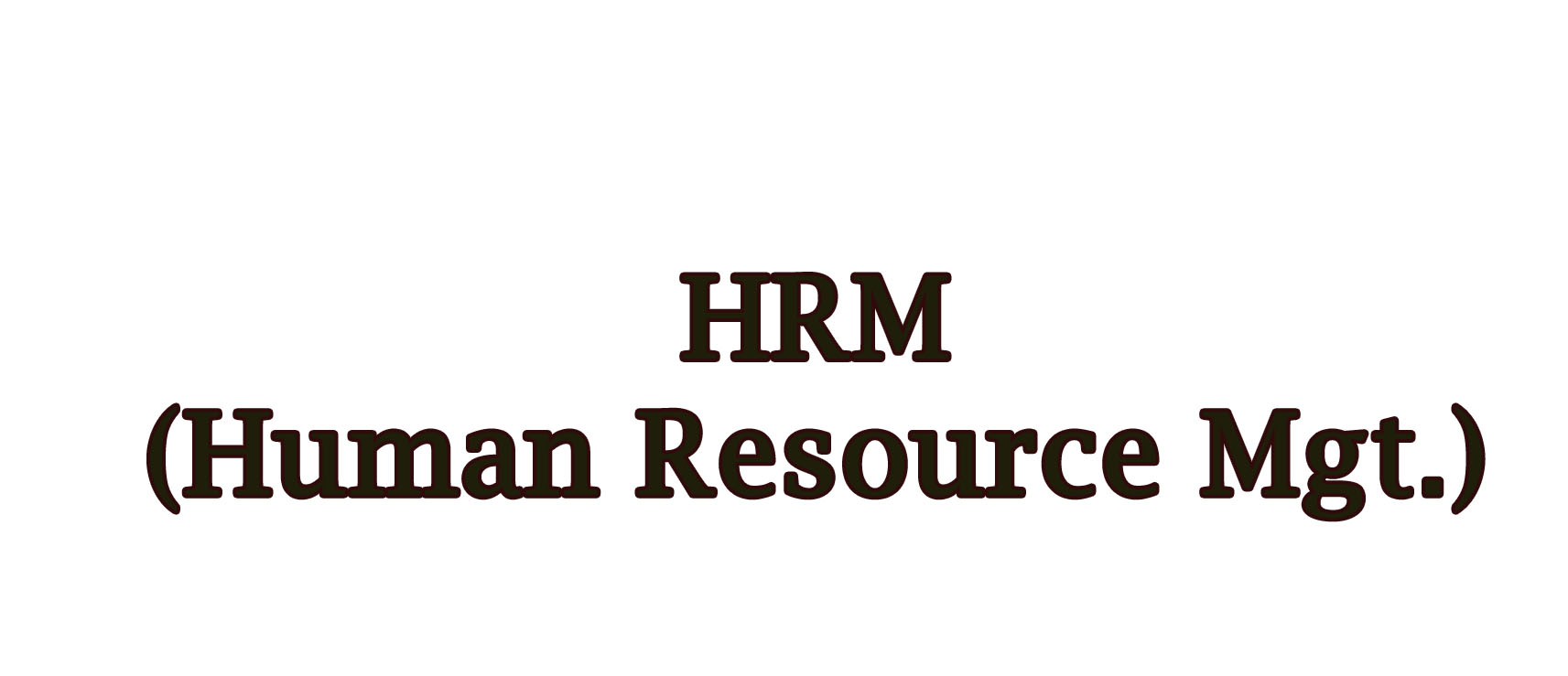HRM

Our BrochuresHRM

HRM Important Question PDF

For  (Eco + HRM) @ 299 Rs.
For   (Eco + HRM + Computer) @ 399 Rs.

Pay by Debit/credit Card/Net Banking  Or Paytm (Secure)

Find Available

OUR PDF DocumentsQUESTION 1 (ENG.)

QUESTION 1 (ENG.)IMPORTANT QUESTION HRM

IMPORTANT QUESTION HRMIMPORTANT QUESTION HRM SAMPLE PAPER 1

IMPORTANT QUESTION HRM SAMPLE PAPER 1IMPORTANT QUESTION HRM SAMPLE PAPER 2

IMPORTANT QUESTION HRM SAMPLE PAPER 2IMPORTANT QUESTION HRM SAMPLE PAPER 3

IMPORTANT QUESTION HRM SAMPLE PAPER 3QUESTION 2 (ENG.)

QUESTION 2 (ENG.)QUESTION 3 (ENG.)

QUESTION 3 (ENG.)QUESTION 4 (ENG.)

QUESTION 4 (ENG.)QUESTION 5 (ENG.)

QUESTION 5 (ENG.)QUESTION 6 (ENG.)

QUESTION 6 (ENG.)QUESTION 8 (ENG.)

QUESTION 8 (ENG.)QUESTION 9 (ENG.)

QUESTION 9 (ENG.)QUESTION 1 (HINDI.)

QUESTION 1 (HINDI.)QUESTION 2 (HINDI.)

QUESTION 2 (HINDI.)QUESTION 3 (HINDI.)

QUESTION 3 (HINDI.)QUESTION 4 (HINDI.)

QUESTION 4 (HINDI.)QUESTION 5 (HINDI.)

QUESTION 5 (HINDI.)QUESTION 6 (HINDI.)

QUESTION 6 (HINDI.)QUESTION 8 (HINDI.)

QUESTION 8 (HINDI.)QUESTION 9 (HINDI.)

QUESTION 9 (HINDI.)Find Available